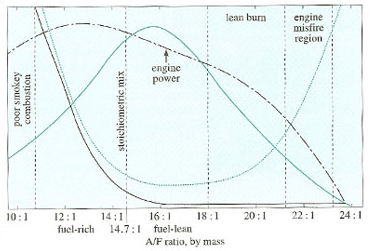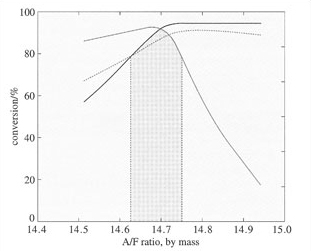The three-way catalytic converter

Start this free course now. Just create an account and sign in. Enrol and complete the course for a free statement of participation or digital badge if available.

Free course

# 4.3 Exhaust emission characteristics

Before we consider how the three-way catalyst functions in any detail, it is important to understand how the emissions of CO, HC and NOx, from the engine depend on the ratio of air (A) to fuel (F) – the air/fuel ratio (or A/F ratio). The significance of this will become clear when we see that the ratio at which the three-way catalytic converter operates is crucial for its success.

## SAQ 1

Taking octane (C8H18) to be the only constituent of fuel, and assuming that air is 20% O2 by volume, estimate the stoichiometric A/F ratio (mass ratio) required for total combustion to occur. At this stage neglect the effect of NO as an oxidant. Comment on the difference between the value you obtain and the experimental value of 14.7:1 (Use the following relative atomic masses: C, 12.01; H, 1.01; O, 16.00; N, 14.01.)

The stoichiometric equation for the complete combustion of octane can be written as follows:

C8H18 + 121/2O2 = 8CO2 + 9H2O

So combustion of 1 mol of octane will require 12.5 mol of oxygen.

Assuming air to be approximately 20% O2 and 80% N2 (by volume), the mass of air required will be 12.5 (32.00 + 4 × 28.02) g = 1 801 g.

The mass of 1 mol of octane is (8 × 12.01 + 18 × 1.01) g = 114.26 g.

Thus, the A/F mass ratio for complete combustion is:

A/F = 1 801/114.26

= 15.8:1

This is as close as you would expect to the experimental value of 14.7:1, because we have used a very simplified system. We had not included NO as an oxidant or the other hydrocarbons, CO or H2 as reductants, and we have used octane, not the real mix of hydrocarbons in petrol.Figure 5 The effect of changing air/fuel ratio on the levels of NOx (solid green), CO (black) and HC (dotted green) produced in the engine. The diagram also shows qualitatively how the engine power output changes with the A/F ratio.

A general relationship between levels of CO, HC and NOx released from the engine and the A/F ratio is shown in Figure 5. At A/F ratios somewhat above stoichiometric (14.7:1) – that is, when the engine is operating under fuel-lean, net oxidising conditions – low levels of HC and CO are produced in the engine, and there is a peak in NOx concentration. At higher A/F values, NOx falls, but the hydrocarbon concentration increases as the engine begins to misfire.

## Activity 1

Why do you think the CO and HC levels in Figure 5 increase under fuel-rich conditions; that is, at low A/F ratios?

### Discussion

The levels released in the engine increase because, below the stoichiometric ratio, there is insufficient oxygen present for total combustion

When the exhaust gas is close to its stoichiometrically balanced composition, at an A/F ratio of about 14.7:1, the concentrations of oxidising gases (NO and O2) and reducing gases (HC and CO) are matched; in theory, it should then be possible to achieve complete conversion to produce only CO2, H2O and N2. This is, of course, the objective of the three-way catalytic converter, and so, ideally, it should be operated in a narrow band, or window, close to the stoichiometric ratio, within which it will promote simultaneously the nearly complete reduction of NOx to N2 and the nearly complete oxidation of CO and HC to CO2 and H2O. Figure 6 shows the catalyst conversion efficiency for all three classes of pollutants as a function of A/F ratio, with the dotted lines defining the window for conversions of 80% and above.Figure 6 Activity of a three-way catalyst for the simultaneous conversion of NOx (solid green), CO (black) and HC (dotted green) as a function of the air/fuel ratio. The shaded area defines the window for conversions of 80% and above for all three pollutants. (Note that, for clarity, the A/F ratios are expressed as the amount of air per unit of fuel, e.g. 14.7 instead of 14.7 : 1. We shall use this notation for the rest of this course.)

## Activity 2

Ideally, would we want this window to be as wide or as narrow as possible?

### Discussion

A wide window is desirable for catalytic emission control as it lessens the need to tighten the A/F control of the engine.

This window also happens to correspond closely to the optimum range for high performance of the vehicle (see engine power in Figure 5), which was also of growing importance at the time of development (1970s).

## Study comment

You should approach the following SAQ by thinking about how the mixture expelled from the engine will vary depending on the A/F ratio, and the effect that this will have on the balance of reductants/oxidants present. You should consider how efficient the catalyst will be in converting this mixture, and hence, how this will affect the gases finally emitted from the exhaust (that is, leaving the catalyst).

## SAQ 2

Using the information given in Figures 5 and 6, explain the changes in conversion efficiency seen for all three pollutants when the A/F value is (a) greater than the window for optimum conversion, and (b) less than the window for optimum conversion.

(a) Figure 5 shows that over the narrow range of A/F ratios covered in Figure 6 the amounts of CO and HC emitted form the engine decrease as A/F increases. As there is a simultaneous increase in the total amount of oxidants (air + NOx), the overall conversion of CO and HC increases to approach effective completion at the stoichiometric ratio, and then remains constant in the net oxidising conditions beyond that point. The sharp fall in NOx conversion for A/F values approaching and above stoichiometric is understandable in terms of the virtual elimination of reductants in this region. Because the system is unable to remove all of the NOx, we would expect to see an increase in NOx emissions from the exhaust. The three-way catalytic converter is therefore unsuitable for engines that run lean.

(b) At A/F ratios below the window value there is less NOx and more HC and CO present in the mixture expelled from the engine (Figure 5). All the NOx present will react over the catalyst, so the NOx conversion will still be high (as seen in Figure 6). However, we see a decrease in catalyst efficiency for destroying HC and CO, as there are insufficient oxidants present for complete conversion. We would therefore expect to see an increase in the HC and CO levels emitted from the exhaust.

Obviously in both cases, in the absence of the three-way converter the levels of CO, HC and NOx emitted for the exhaust would be much higher.

Engine control systems have been developed to include an oxygen sensor (or lambda, λ, sensor as it is sometimes called), and an electronic module to regulate the A/F ratio, so that the exhaust composition is kept within the window for optimum conversion. However, because there are time delays in the A/F correction, the ratio cycles very rapidly between slightly fuel-rich and slightly fuel-lean, oscillating about the stoichiometrically balanced composition (14.7 ± 0.3) at a typical frequency of 1 cycle per second. Minimising the amplitude of the oscillation increases the effectiveness of the converter.

S342_1